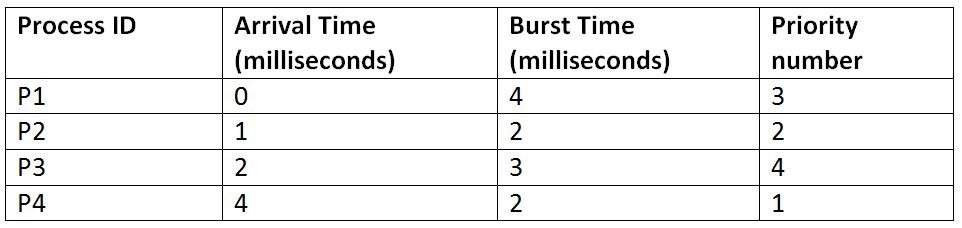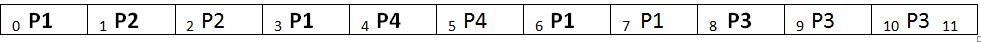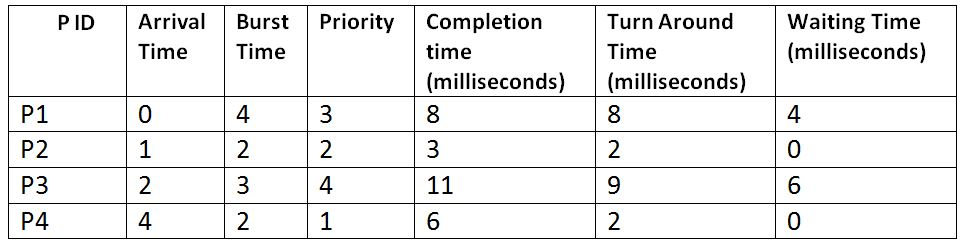# Priority Scheduling (Preemptive) in Operating System

In this article, we are going to learn about the preemptive Priority scheduling, which is a type of process scheduling algorithms in the Operating systems. We will first describe how the processes are scheduled inside the system by using this algorithm and then will look at an example for the further understanding of the entire working process of the operating system while following this scheduling algorithm.
Submitted by Monika Sharma, on June 24, 2019

In the priority scheduling, the processes are scheduled on the basis of their priority, and not on the basis of their burst time. If the preemptive mode of this scheduling is being followed, then a process with a higher priority than the currently executing process can replace the executing process. This can be well explained with the help of the following example:

Suppose, we have four processes: P1, P2, P3 and P4, and they enter the CPU in the following manner:As per the preemptive priority scheduling, the processes will be executed as follows:

Gant ChartExplanation:

• There is only P1 available at time 0, so it will be executed first irrespective of the priority until some other process with a higher priority is encountered by the OS.
• At the beginning of the 1st-time unit, we have P2 which has a higher priority than P1, so it replaces P1.
• At 2nd time unit, we have the process P3, but its priority is less than the executing process P2, so P2 keeps executing without any replacement.
• At 3rd time unit, our P2 has been completed and till now we have processes P1 and P3 left. So, P1 which has a higher priority than P3 is executed.
• At 4th time unit, we have process P4 which has the highest priority of them all. So, it replaces P1.
• Since now we have no more processes left to arrive, the processes will now run according to their priorities and will complete their execution.```    Total Turn-Around Time = 8 + 2 + 9 + 2
= 21 milliseconds
Average Turn-Around Time= Total Turn-Around Time / Total No. of Processes
= 21 / 4
= 5.25 milliseconds

Total Waiting Time = 4 + 0 + 6 + 0
= 10 milliseconds
Average Waiting Time = Total Waiting Time / Total No. of Processes
= 10 / 4
= 2.5 milliseconds
```